# Compare - Definition with Examples

The Complete Prek-5 Learning Program Built for Your Child
Home > Compare

## What is Compare?

The dictionary definition of compare (verb) is to view something in relation to another. In math, to compare means to examine the differences between numbers, quantities or values to decide if it is greater than, smaller than or equal to another quantity.

Here, for instance, we are comparing numbers.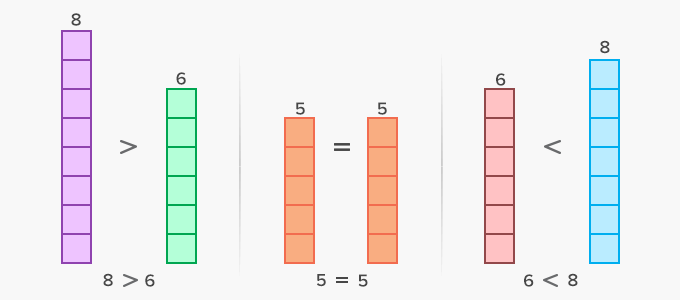By comparing, we can define or find by how much a number is greater or smaller.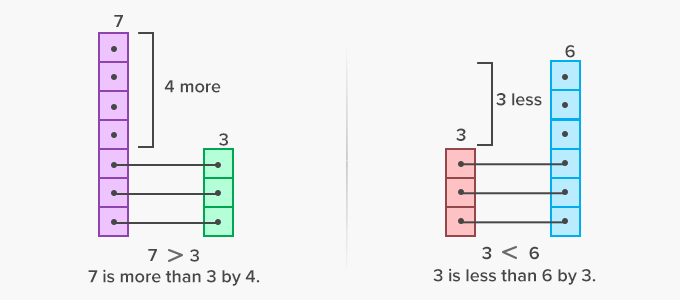Here’s how we can also compare decimals and fractions.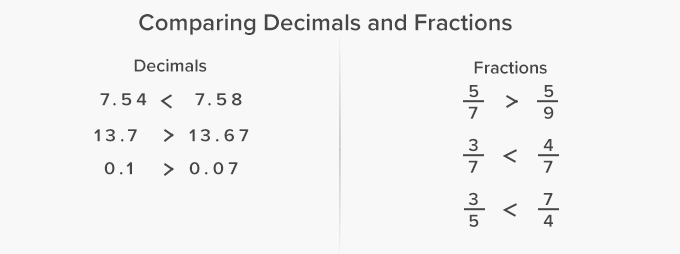Here’s how we can make comparisons in our daily life.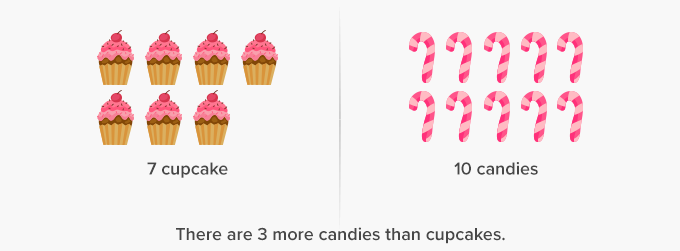Here, we are comparing the length of two line segments.We can also compare distances between two places.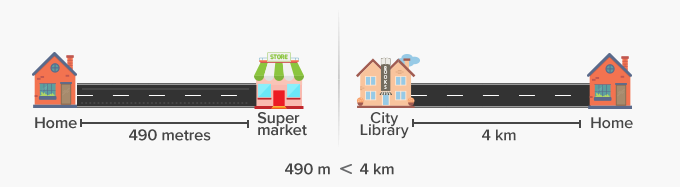Here’s how we can make compare two quantities in terms of their weight.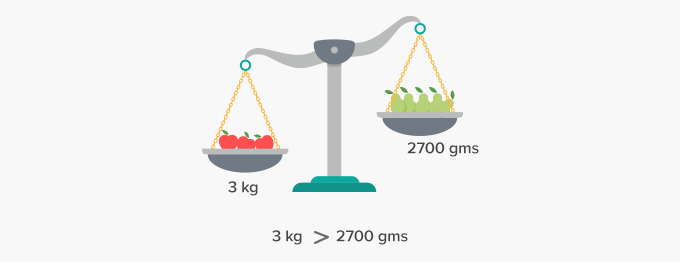Here, we are comparing the volume of liquids.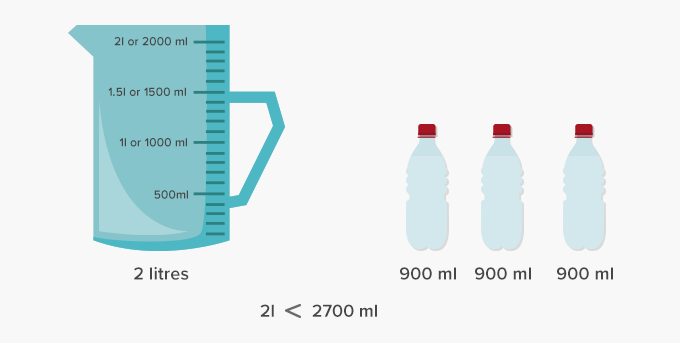Fun Facts We can also compare how shapes are alike or different.

##Let's do it!

Instead of handing out comparison worksheets, ask your child to compare different things around him, for instance, the number of cookies in two jars or the quantity of juice in two glasses.

##Related math vocabulary

Estimate